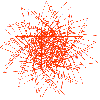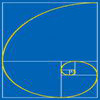# Search by Topic

#### Resources tagged with Fibonacci sequence similar to Fibonacci Fashion:

Filter by: Content type:
Age range:
Challenge level:

### There are 21 results

Broad Topics > Patterns, Sequences and Structure > Fibonacci sequence### Fibonacci Fashion

##### Age 16 to 18 Challenge Level:

What have Fibonacci numbers to do with solutions of the quadratic equation x^2 - x - 1 = 0 ?### Gnomon Dimensions

##### Age 14 to 16 Challenge Level:

These gnomons appear to have more than a passing connection with the Fibonacci sequence. This problem ask you to investigate some of these connections.### Plus or Minus

##### Age 16 to 18 Challenge Level:

Make and prove a conjecture about the value of the product of the Fibonacci numbers $F_{n+1}F_{n-1}$.### Pythagorean Fibs

##### Age 16 to 18 Challenge Level:

What have Fibonacci numbers got to do with Pythagorean triples?### Fibonacci Factors

##### Age 16 to 18 Challenge Level:

For which values of n is the Fibonacci number fn even? Which Fibonnaci numbers are divisible by 3?### Farey Fibonacci

##### Age 16 to 18 Short Challenge Level:

Investigate Farey sequences of ratios of Fibonacci numbers.### Ordered Sums

##### Age 14 to 16 Challenge Level:

Let a(n) be the number of ways of expressing the integer n as an ordered sum of 1's and 2's. Let b(n) be the number of ways of expressing n as an ordered sum of integers greater than 1. (i) Calculate. . . .### Leonardo of Pisa and the Golden Rectangle

##### Age 7 to 16

Leonardo who?! Well, Leonardo is better known as Fibonacci and this article will tell you some of fascinating things about his famous sequence.### Golden Mathematics

##### Age 16 to 18

A voyage of discovery through a sequence of challenges exploring properties of the Golden Ratio and Fibonacci numbers.### Golden Fibs

##### Age 16 to 18 Challenge Level:

When is a Fibonacci sequence also a geometric sequence? When the ratio of successive terms is the golden ratio!### Building Gnomons

##### Age 14 to 16 Challenge Level:

Build gnomons that are related to the Fibonacci sequence and try to explain why this is possible.### Got a Strategy for Last Biscuit?

##### Age 11 to 16 Challenge Level:

Can you beat the computer in the challenging strategy game?### Continued Fractions I

##### Age 14 to 18

An article introducing continued fractions with some simple puzzles for the reader.### Golden Powers

##### Age 16 to 18 Challenge Level:

You add 1 to the golden ratio to get its square. How do you find higher powers?### Golden Fractions

##### Age 16 to 18 Challenge Level:

Find the link between a sequence of continued fractions and the ratio of succesive Fibonacci numbers.### The Golden Ratio, Fibonacci Numbers and Continued Fractions.

##### Age 14 to 16

An iterative method for finding the value of the Golden Ratio with explanations of how this involves the ratios of Fibonacci numbers and continued fractions.### First Forward Into Logo 11: Sequences

##### Age 11 to 18 Challenge Level:

This part introduces the use of Logo for number work. Learn how to use Logo to generate sequences of numbers.### Spirals Instead of Sunflowers

##### Age 11 to 16 Challenge Level:

Using logo to investigate spirals### LOGO Challenge - Circles as Bugs

##### Age 11 to 16 Challenge Level:

Here are some circle bugs to try to replicate with some elegant programming, plus some sequences generated elegantly in LOGO.### Whirling Fibonacci Squares

##### Age 11 to 16

Draw whirling squares and see how Fibonacci sequences and golden rectangles are connected.### Stringing it Out

##### Age 14 to 16 Challenge Level:

Explore the transformations and comment on what you find.